Courses

# Basic Concepts (Structure Of Solids), Hardness Test & Crystalline Materials - MCQ Test 2

## 30 Questions MCQ Test RRB JE for Mechanical Engineering | Basic Concepts (Structure Of Solids), Hardness Test & Crystalline Materials - MCQ Test 2

Description
This mock test of Basic Concepts (Structure Of Solids), Hardness Test & Crystalline Materials - MCQ Test 2 for Mechanical Engineering helps you for every Mechanical Engineering entrance exam. This contains 30 Multiple Choice Questions for Mechanical Engineering Basic Concepts (Structure Of Solids), Hardness Test & Crystalline Materials - MCQ Test 2 (mcq) to study with solutions a complete question bank. The solved questions answers in this Basic Concepts (Structure Of Solids), Hardness Test & Crystalline Materials - MCQ Test 2 quiz give you a good mix of easy questions and tough questions. Mechanical Engineering students definitely take this Basic Concepts (Structure Of Solids), Hardness Test & Crystalline Materials - MCQ Test 2 exercise for a better result in the exam. You can find other Basic Concepts (Structure Of Solids), Hardness Test & Crystalline Materials - MCQ Test 2 extra questions, long questions & short questions for Mechanical Engineering on EduRev as well by searching above.
QUESTION: 1

Solution:

Ans. (a)

QUESTION: 2

Solution:

Ans. (b)

QUESTION: 3

### Assertion (A): Elements are classified into metals and non-metals on the basis oftheir atomic weights. Reason (R): The valence electron structures contribute to the primary bonding between the atoms to form aggregates.

Solution:

Ans.(d)

QUESTION: 4

Which of the following would you prefer for checking the hardness of very thinsections?

Solution:

Ans. (c)

QUESTION: 5

Assertion (A): Unlike in the case of ionic bonds, the co-ordination numbers for covalently bonded atoms are not controlled by the radii ratio.
Reason (R): A covalent bond has a specific direction of bonding in space.

Solution:

Ans. (c)

QUESTION: 6

Magnesium is extruded and not rolled because

Solution:

Ans. (a)

QUESTION: 7

With the increase of percentage of carbon in the steel, which one of the following properties does increase?

Solution:
QUESTION: 8

For a Rhombohedral space lattice, which one of the following is correct?

Solution:

Ans. (b)

QUESTION: 9

Which one of the following pairs is not correctly matched?
Space Lattice          Relation between Atomic radius r and Edge element a

Solution:

Ans. (c)

QUESTION: 10

Match List-I (Name of the Element) with List-II (Crystal Structure) and select the correct answer using the codes given below the lists:
List I                                                          List II
A. Fluorspar                                    1. Body-centered cubic
B. Alpha-Iron                                   2. Hexagonal closed packed
C. Silver                                          3. Simple cubic
D. Zinc                                            4. Face-centered cubic
Codes: A B C D                      A B C D
(a) 3 2 4 1                   (b) 4 1 3 2
(c) 4 2 3 1                   (d) 3 1 4 2

Solution:

Ans. (d)

QUESTION: 11

Match List-I (Element) with List-II (Crystal Structure) and select the correct answer using the code given below the Lists:
List - I                                                     List - II
A. Alpha Iron                                     1. Hexagonal closed packed
B. Copper                                          2. Body-centred cubic
C. Zinc                                               3. Amorphous
D. Glass                                            4. Face-centred cubic
Codes: A B C D                             A B C D
(a) 2 3 1 4                          (b) 1 4 2 3
(c) 2 4 1 3                          (d) 1 3 2 4

Solution:

Ans. (c)

QUESTION: 12

In the atomic hard-sphere model of the crystal structure of Copper, what is the edge length of unit cell?

Solution:

Ans. (c)
Cu – F.C.C Structure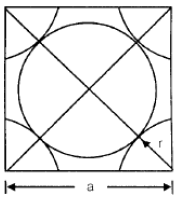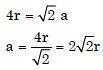Edge length of unit all = (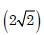) × Atomic radius.

QUESTION: 13

The coordination number for FCC crystal structure is

Solution:
QUESTION: 14

Assuming atoms to be perfect spheres, what is the value of the highest possible atomic packing factor (APF) in metals?

Solution:

Ans. (b) Packing factor = 0.74 which is maximum and for F.C.C and HCP structure.

QUESTION: 15

Which one of the following is correct for 'Climb'?

Solution:

Ans. (b) Climb is a dislocation movement in which dislocation moves from one slip plane to another slip plane. Where as Glide is a dislocation movement in which dislocation moves within the same slip plane.

QUESTION: 16

What is the movement of block of atoms along certain crystallographic planes and directions, termed as? [IES 2007]

Solution:

Ans. (c)

QUESTION: 17

What is a surface imperfection, which separates crystals of different orientationsin a poly-crystalline aggregate, called? [IES-2008]

Solution:

Ans. (c) There are several types of planar (or surface) defects that occur from a change in the orientation of crystallographic planes across a surface boundary. The most important planar defect is the grain boundary, which is the imperfect plane surface that separates two crystals of different orientation in a polycrystalline solid. Grain boundaries originate when the last few remaining atoms of a liquid freeze onto the meeting faces of two adjacent crystals that have grown from the melt or, similarly, when two adjacent crystals that grow
by re-crystallization meet each other.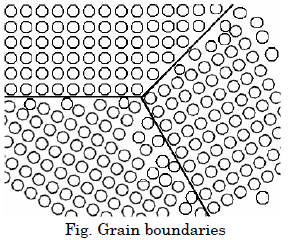QUESTION: 18

Assertion (A): Refining the grain size of a polycrystalline material renders itharder and stronger.
Reason (R): Grain boundaries provide easy paths to dislocation motion.

Solution:

Ans. (c)

QUESTION: 19

Which one of the following is correct for "Burger's vector" in screw dislocation?

Solution:

Ans. (c) In screw dislocation Burger Vector is parallel to the dislocation line whereas in
Edge dislocation, dislocation is perpendicular to the dislocation line.

QUESTION: 20

Chemicals attack atoms within grain boundaries preferentially because they have

Solution:

Ans. (b)

QUESTION: 21

What is the approximate strain energy expression for a dislocation of unit length, irrespective of its edge or screw character?

Solution:

Ans. (b)

QUESTION: 22

A screw dislocation
1. Lies parallel to its Burger's vector
2. Lies perpendicular to its Burger's vector
3. Moves in a perpendicular direction to the Burger's vector
4. Moves in an inclined direction to the Burger's vector
Select the correct answer using the codes given below:
Codes:

Solution:

Ans. (b)

QUESTION: 23

Which one of the following statements is correct in the case of screw dislocations?(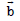= Burgers Vector;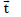= Imaginary Vector)

Solution:

Ans. (b) Burger vector ()  is parallel to imaginary vector () in case of screw dislocation.

QUESTION: 24

Which of the following factors govern solubility of two non-ferrous metals both inliquid state, as well as in solid state?
1. Crystal structure
2. Relative size factor
3. Chemical-affinity factor
4. Relative valence factor
Select the correct answer using the codes given below:
Codes:

Solution:

Ans. (d)

QUESTION: 25

Which one of the following pairs is not correctly matched?

Solution:

Ans. (b)

QUESTION: 26

Assertion (A): Carbon forms interstitial solid solution when added to iron.Reason (R): The atomic radius of carbon atom is much smaller than that of iron.

Solution:

Ans. (a)

QUESTION: 27

Which one of the following defects is 'Schottky defect’?

Solution:

Ans. (a) Schottky defect is a type of vacancy defect in which cation vacancy is associated with anion vacancy.

QUESTION: 28

Which of the following is a point imperfection?
1. Vacancy
2. Interstitialcy
3. Frenkel imperfection
4. Schottky imperfection

Solution:

Ans. (d)

QUESTION: 29

Consider the following statements about FCC and HCP crystal structure:
1. Both have same coordination number and atomic packing fraction.
2. Both represent closely packed crystal structures.
3. Both structures are generated by stacking of close packed plants on top of one another,but only the stacking sequence is different.
Which of the statements given above are correct?

Solution:

Ans. (d) Both have same co-ordination number 12 and atomic packing fraction 0.74.

QUESTION: 30

Which of the following properties of a solid are dependent on crystal imperfecttions?
1. Yield stress
2. Melting point
3. Semiconductivity
4. Ductility
Select the correct answer using the codes given below:

Solution:

Ans. (b)